Open in App
Not now

# How To Hide Message or Image Inside An Image In MATLAB?

• Last Updated : 19 Oct, 2022

MATLAB is a high-performance language that is used for matrix manipulation, performing technical computations, graph plottings, etc. It stands for Matrix Laboratory. With the help of this software, we can also hide a message or image inside an image. Following are the steps to hide an image, which contains some text, inside the image. Then we’ll extract the hidden text also.

## How To Hide Image or Message Inside An Image:

Each pixel of an image stores color in binary form. We change one of the planes with our binary message image so that we can transmit it secretly and the receiver can extract the message.

### Method To Hide a Secret Text Image In An Image: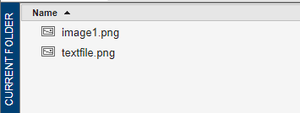images before performing the steps

Example 1:

## Matlab

 `% MATLAB code for Hide Secret Text in Image``% Read the image``  ``original = imread(``'7.png'``);``  ``imshow(original);title(``'Original Image'``);` `% Import the secret message image``% and convert it to binary image``  ``secretmessage = imread(``'textfile.png'``);``  ``msg = imbinarize(rgb2gray(secretmessage));` `% Resize the original image and secret``% message image to same size``    ``msg = imresize(msg,size(original(:,:,1)));` `% Select a bit plane and change it to our message``    ``temp = original;` `% Changing 'r' plane and using least significant bit(1)``% so that the image remains hidden``    ``temp(:,:,1) = bitset(temp(:,:,1),1,msg);` `% Saving the image``  ``figure,imshow(temp);title(``'Encrypted Image'``);``  ``imwrite(temp,``'encryptedImage.bmp'``);`

Output:

Image showing that ‘encryptedImage.bmp’ has been created: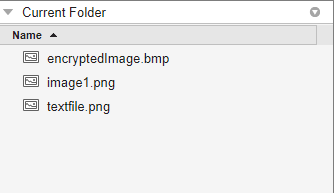Explanation:

1. Read the original image and show it with the title ‘Original Image’.
2. Now we’ll read the secret message image and convert it to a binary image. To convert it to binary we have to first convert it to gray.
3. Now we’ll resize the original image and secret message image to the same size as the originals.
4. Now select a bit plane and change it to the secret message. Here we are changing the red (r) plane. Also, use the least significant bit(1) so that the image remains hidden.
5. Now show the image in figure2 and save the image. Here we are saving the image in .bmp format because it will store the image in an uncompressed form and hence the bits will not change.

So, Now our image ‘encryptedImage.bmp’ is having the secret message image hidden in it.

### Method To Extract a Secret Text Image From An Image:

Here we method to extract a secret text image from an image using the following code.

Example 2:

## Matlab

 `% MATLAB code extract message from an image``% Read encrypted image``    ``img = imread(``'encryptedImage.bmp'``);` `% Extract the bitplane of the hidden message``    ``hiddenMessageImage = bitget(img(:,:,1),1);` `% Showing the image``    ``figure,imshow(logical(hiddenMessageImage));title(``'Secret Text'``);`

Output:

Original Image: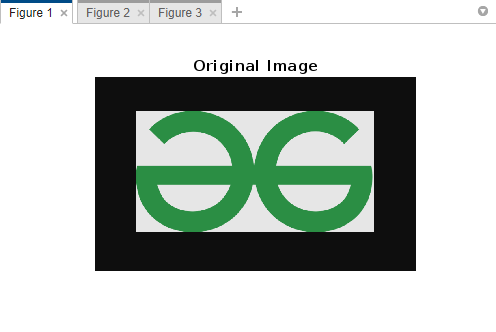An image containing the secret message: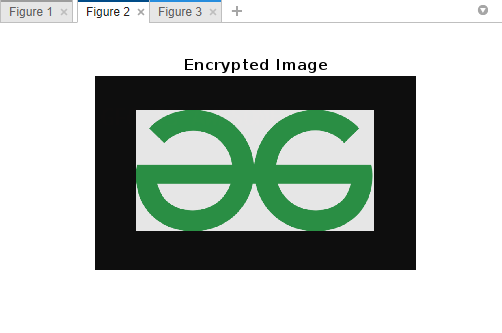Secret Text: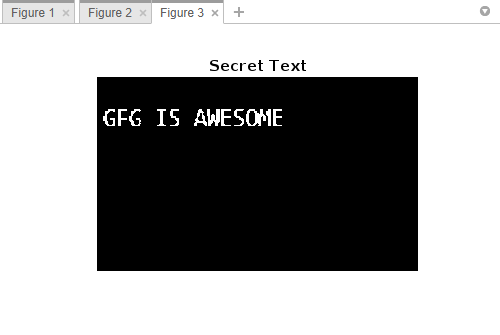Explanation:

1. Read the encrypted image and extract the bitplane of the hidden message.
2. Show the secret text in figure3 by using imshow().

My Personal Notes arrow_drop_up